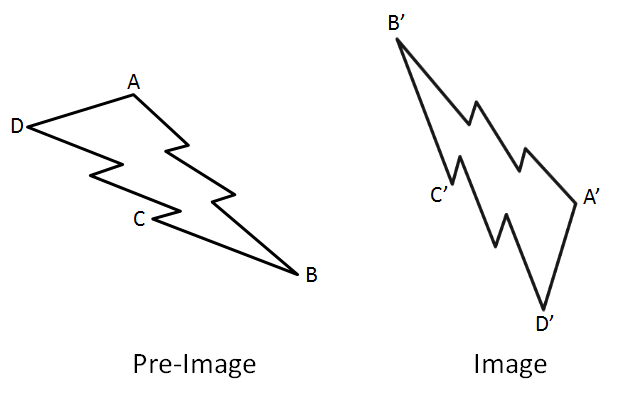# Transformations – Three Sequences

Directions: List three sequences of transformations that take pre-image ABCD to image A’B’C’D’.### Hint

What transformations do you need to use? Can you use the same transformations and do it another way?

There are an infinite number of answers but they all must involve a reflection. Most will also include a rotation (but not necessarily).

Source: Robert Kaplinsky

## Equations of Circles 1

Directions: Using the integers -9 to 9, at most one time each, fill in the …

1.reflect Shape ABCD on a virticle line in the middle of the two shapes then rotate shape ABCD off point D than tranform point D to point D prime

2.rotate 360 clockwise. Reflect on AD.
Translate up 2. Reflect on AD

3.Rotate shape ABCD 180 degrees then reflect shape ABCD on a middle line between shape ABCD and shaper ABCD prime then translate point D from shape ABCD to point D’ on shape ABCD prime.

4.Reflect over vertical line in between the shapes, rotate 90 degrees clockwise.

5.Reflection between the middle of the pre-image and image, and rotate 180degree at the middle point.

6.Some transformations that will bring shape ABCD to ABCD prime, is a reflection of shape ABCD in the middle if the two shapes (vertical), then a rotation 90 degrees clockwise, then a translation from point D to point D prime